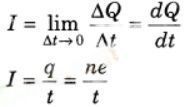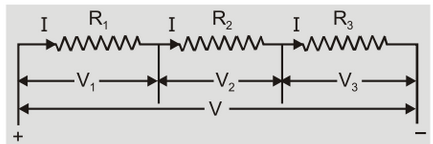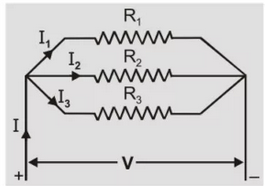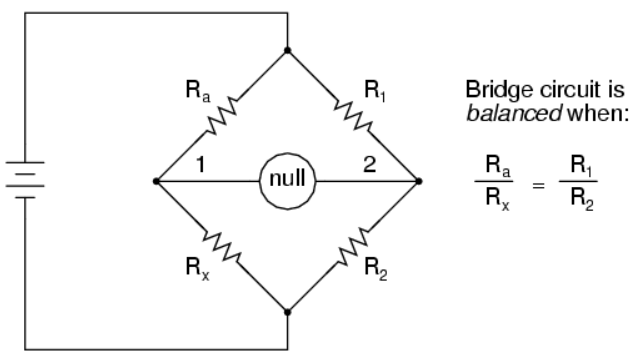# Current Electricity

## Current Electricity class 12th physics

### Electric Current

The directed rate of flow of electric charge through any cross-section of a conductor is known as electric current.
If ∆Q charge flows in time ∆t, then current at any time t is[q = ne]

### Ohm's Law

If V is in volt, I in ampere and R is in ohm, R is the electrical resistance of the conductor.
V  ∝ I### Specific Resistance

ρ of a conductor is given by, R = ρL/A, where A is the area of cross section and L, the length of the conductor. If R is in ohm, A in m2 and L in m, ρ is in ohm-metre,

### Variation Of Resistance With Temperature

Temperature and resistance: α is the temperature coefficient of resistance.

### (a) Resistances in series(b) Resistances in parallel### Joule's Law

Heat produced in a conductor of resistance R, when a current I flows for t seconds = I2 Rt joules

### Power In A Circuit

P = ΔW/Δt

P = IV

By Ohm's Law, V = IR,

P = I2R = V2/R

### Kirchhoff's Laws

(a) The sum of the currents that flow into a junction is equal to the sum of the currents that flow out of the junction.

(b) The sum of the emf’s around a loop is equal to the sum of the potential drops around the loops.
In writing V = IR  = 0, the current into a node (or junction) is taken to be positive.
In applying Kirchhoff’s voltage law, if there are more than one source when the directions do not agree, the voltage of source is taken as positive, if it is in the direction of assumed current.

### Wheat Stone Bridge (Applied To Meter Bridge And For Capacitor Also )

For null deflection in